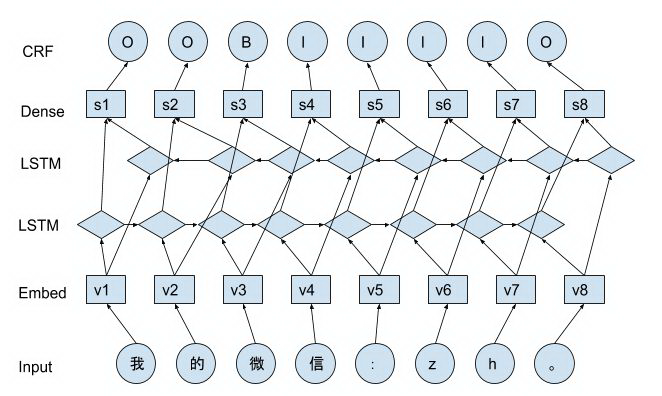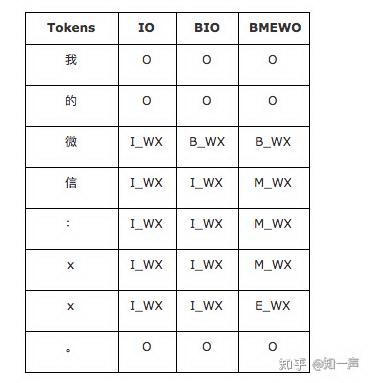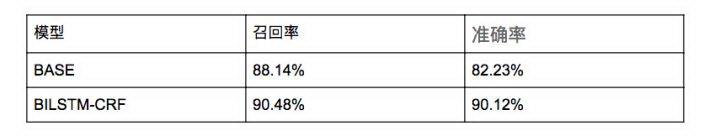# 算法在社区氛围的应用（一）：识别垃圾广告导流信息@知乎小管家 也在自己的专栏中进行了整体地介绍了算法最新的进展： 瓦力解锁新技能：实时识别处理多种违规行为。其实，知一声也邀请了几位小伙伴来从技术的角度分享算法识别背后的原理。

## 网络结构

BILSTM-CRF 模型结构如下图所示。BILSTM-CRF 模型同时结合了 LSTM 和 CRF 的优点，使得其在序列标注任务上具有极强的优势。

## CRF

$P(y|x)={1\over Z(x)}\prod\limits^T_{t=1}exp\left{\sum\limits^K_{k=1}\theta_kf(y_{t-1},y_t,x)\right}$

$Z(x)=\sum\limits_y\prod\limits^T_{t=1}exp\left{\sum\limits^K_{k=1}\theta_kf(y_{t-1},y_t,x)\right}$

$f_{k,1}=s_{t,k}y_{t,k}$ 转移特征考虑状态之间的变化趋势

$f_{k,2}=y_{t-1,k}Uy_{t,k}$

## 实体编码

IO 编码是最简单的编码，它将属于类型 X 的实体的序列元素标记为 I_X，不属于任何实体的序列元素标记为 O。这种编码存在缺陷，因为它不能代表彼此相邻的两个实体，因为没有边界标签。

BIO 编码是当前实体编码的行业标准。它将表示实体的 I_X 标签细分为实体开始标签 B_X 和实体延续标签 I_X。

BMEWO 编码进一步区分实体结束元素 E_X 和实体中间元素令 M_X，并为单元素实体添加一个全新的标签 W_X。## 效果